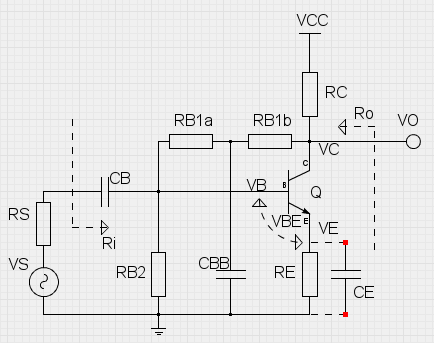OVERVIEW This is a design tool for calculating bias resistor values, small-signal gain and input/output resistances of a common-emitter BJT amplifier with DC feedback for biasing. Just fill the input fields below in given order from top to bottom. The ordering of the fields serves as a step-by-step guide for the design process. The base bias resistor values are calculated automatically (using 5% tolerance resistances), but custom values can be given and those will not be overwritten. The 'Calculate values' button in the bias resistor field always rounds up to the nearest 5% tolerance, even user defined values. The small-signal values are evaluated in the mid-band, CB and CBB short-circuited. CE can be included using the selection box. The small-signal gain is evaluated as VO/VS, and the input/output resistances are evaluated as shown.Select the operating voltage VCC. Typically 9 volts is the way to go. VCC: volts RESULTS DC Bias voltages: VC : volts VE : volts VB : volts AC Voltage Gain factor: Av : const    dB AC Input resistance: Ri : ohms AC Output resistance: Ro : ohms Select the BJT you want to use, from its datasheet lookup the current gain factor and place it here as a parameter. hFE: const For convenience, you can change the base-emitter voltage, which is set to 0.65 V by default. Typical VBE is 0.55 - 0.75 V. VBE: volts Choose the collector bias voltage to aim for. Typically the collector bias voltage is half of the operating voltage VCC. VC: volts The collector resistor RC should be chosen to limit collector current to the suitable level. RC affects base bias and the output impedance. RC : ohms Next select a value of the emitter resistor. RE reduces gain, but it helps to stabilize the DC bias voltages and it also slightly increases the input and output impedances. RE: ohms A multiplier for base resistor values, which are obtained in the next step. Too much resistance at the base affects the stability of biasing. Use values between 2 - 60. Bx: const The biasing is finalized by setting a voltage divider on the base. By pressing the button below, the bias resistor values are calculated automatically. Values have to be cleared before recalculation. RB1a:RB1b:RB2: ohms ohms ohms Also, the internal resistance of the signal source should be given. For ideal source this value is small, but for guitar output this is a few kilohms. RS: ohms Use CE for RE bypass